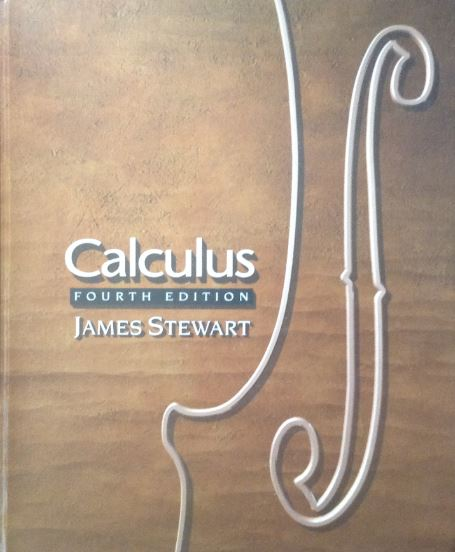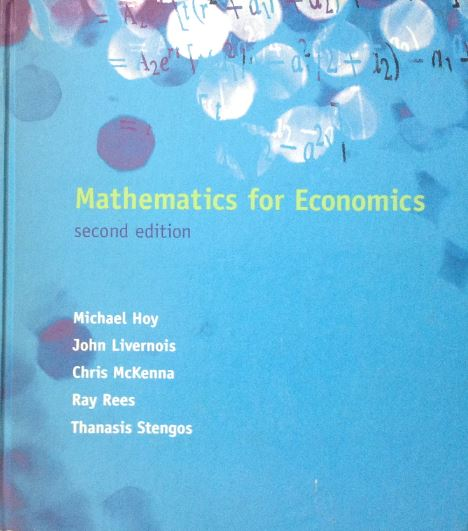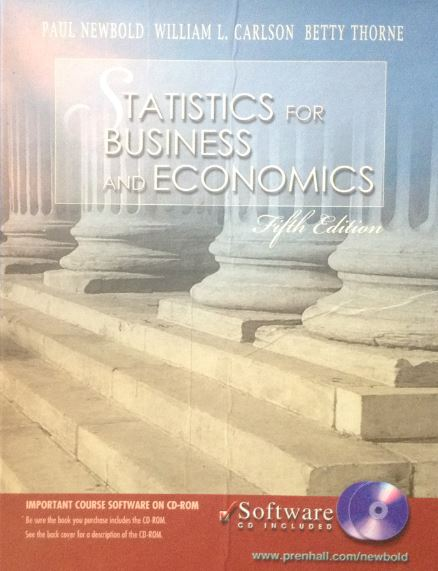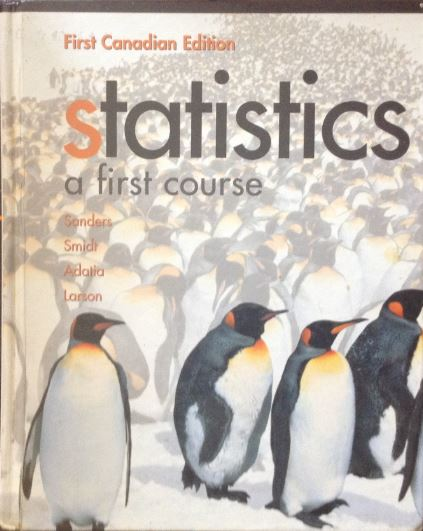# Economics Math & Statistics Tutoring Services

## Hour-long tutoring sessions available through Zoom meetings for Economics Math & Statistics courses• Algebra, Exponents, & Logarithms
• Systems of Linear Equations
• Calculus & Derivative Rules
• Optimization & Drawing Functions## MathematicalEconomicsECON*2770

• Univariate Calculus & Differentiation
• Matrix Operations & Inversion
• Solving Systems of Equations
• Multivariate Constrained Calculus## EconomicStatisticsECON*2740

• Discrete & Continuous Distributions
• Conditional & Unconditional Probabilities
• 5 Steps of Hypothesis Testing
• Confidence Intervals## Intro StatisticsSTAT*2040

• Mean, Median, Mode, & Variance
• Conditional & Unconditional Probabilities
• Types of Continuous & Discrete Distributions
• Hypothesis Testing & Confidence Intervals

Email Tutor@BrightTutoring.ca or book online to schedule a tutoring session now!
Bright Tutoring is the Right Way to Learn!